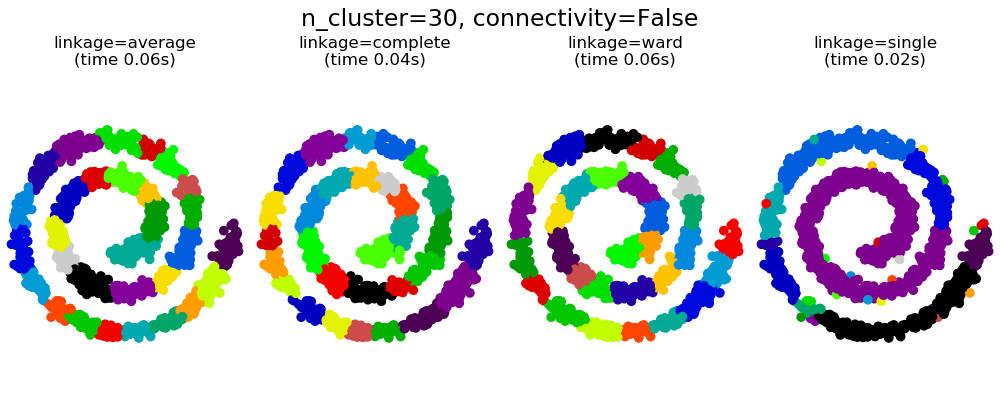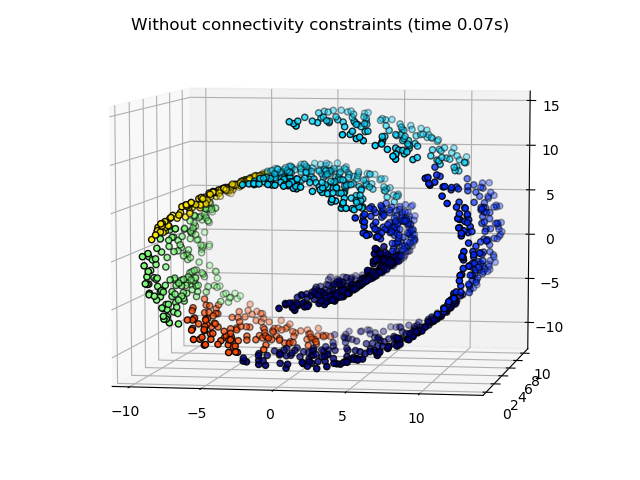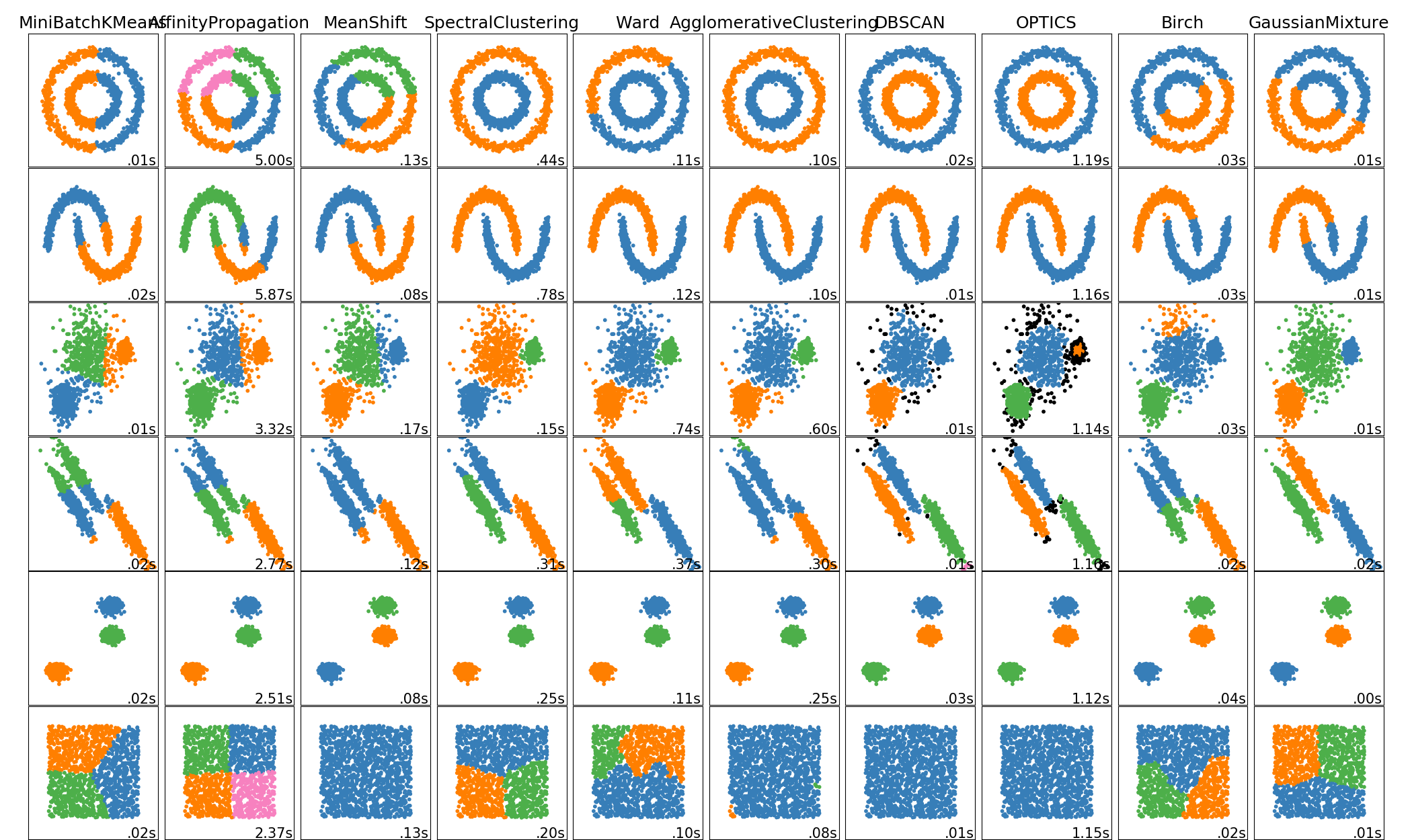# sklearn.neighbors.kneighbors_graph¶

sklearn.neighbors.kneighbors_graph(X, n_neighbors, *, mode='connectivity', metric='minkowski', p=2, metric_params=None, include_=False, n_jobs=None)

[源码]

X array-like of shape (n_samples, n_features) or BallTree

n_neighbors int

mode {‘connectivity’, ‘distance’}, default=’connectivity’

metric str, default=’minkowski’

p int, default=2
Minkowski指标的功率参数。 当p = 1时，这等效于对p = 2使用manhattan_distance（l1）和euclidean_distance（l2）。对于任意p，使用minkowski_distance（l_p）。
metric_params dict, default=None

include_ bool or ‘auto’, default=False

n_jobs int, default=None

A sparse matrix of shape (n_samples, n_samples)

>>> X = [, , ]>>> from sklearn.neighbors import kneighbors_graph>>> A = kneighbors_graph(X, 2, mode='connectivity', include_=True)>>> A.toarray()array([[1., 0., 1.],       [0., 1., 1.],       [1., 0., 1.]])